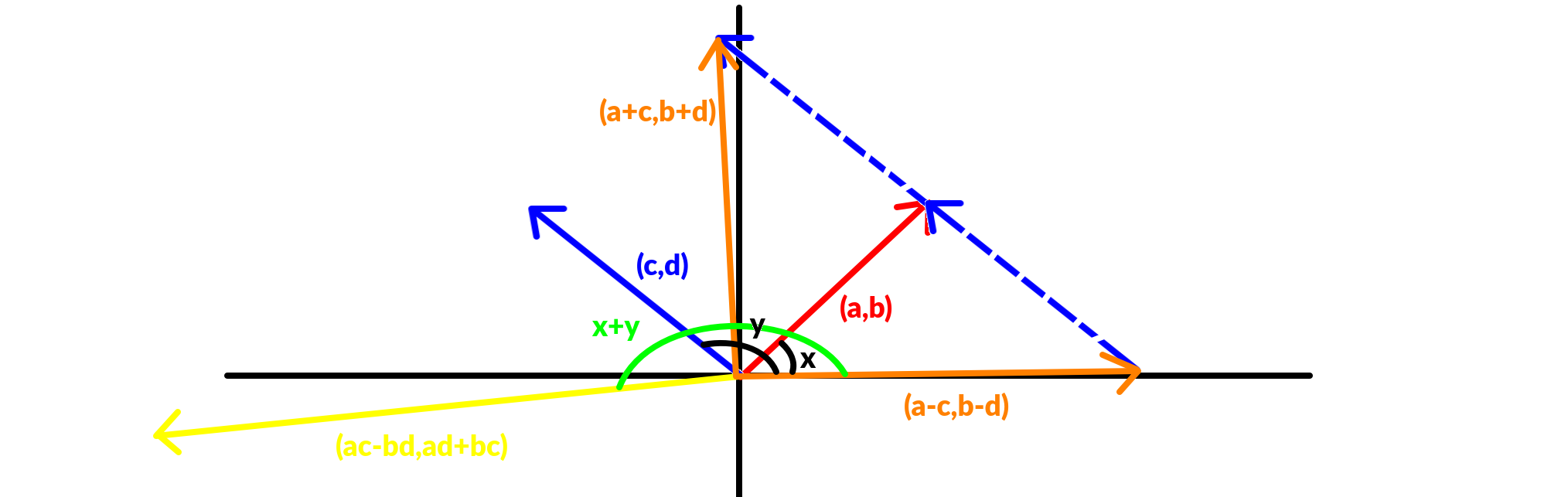复数

复数的运算struct complex{
double a,b;//a+bi
complex(double _a=0,double _b=0){a=_a,b=_b;}//initialize
complex operator+(complex c){return complex(a+c.a,b+c.b);}
complex operator-(complex c){return complex(a-c.a,b-c.b);}
complex operator*(complex c){return complex(a*c.a-b*c.b,a*c.b+b*c.a);}
};

傅里叶变换

FFT 干的事情就是把 次多项式 的系数序列 变换为点值序列 . 当然我们不会保存 的值，所以实际上转换成的序列是

0 000 000 0
1 001 100 4
2 010 010 2
3 011 110 6
4 100 001 1
5 101 101 5
6 110 001 3
7 111 111 7

傅里叶逆变换

#include<cstdio>
#include<algorithm>
#include<cmath>
#include<assert.h>
#define PI M_PI
using namespace std;
const int N=(1<<21)+5;
struct cpx{
double a,b;
cpx(double _a=0,double _b=0){a=_a,b=_b;}
cpx operator+(cpx c){return cpx(a+c.a,b+c.b);}
cpx operator-(cpx c){return cpx(a-c.a,b-c.b);}
cpx operator*(cpx c){return cpx(a*c.a-b*c.b,a*c.b+b*c.a);}
};
int tr[N],dgl;// 蝴蝶变换的下标
void trans(cpx *y,int len){for(int i=1;i<len;i++)if(i<tr[i])swap(y[i],y[tr[i]]);}
void fft(cpx *y,int len,int tag){
trans(y,len);// 蝴蝶变换
for(int j=1;j<len;j<<=1){
cpx wn(cos(PI/j),sin(tag*PI/j));//w_(2*j) 的单位根，2*PI/(2*j)=PI/j
for(int i=0;i<len;i+=j<<1){
cpx w(1,0),u,v;//0 次方
for(int k=i;k<i+j;k++)
u=y[k],v=y[k+j]*w,y[k]=u+v,y[k+j]=u-v,w=w*wn;
}
}
if(tag==-1)for(int i=0;i<len;i++)y[i].a/=len;// 实部
}
int n,m,len=1;
cpx f[N],g[N];
int main(){
scanf("%d%d",&n,&m);
for(int i=0,a;i<=n;i++)scanf("%d",&a),f[i]=cpx(a,0);
for(int i=0,a;i<=m;i++)scanf("%d",&a),g[i]=cpx(a,0);
while(len<m+n+2)len<<=1,dgl++;
for(int i=1;i<len;i++)tr[i]=(tr[i>>1]>>1) | (i&1)<<(dgl-1);// 蝴蝶变换预处理
fft(f,len,1),fft(g,len,1);
for(int i=0;i<len;i++)f[i]=f[i]*g[i];
fft(f,len,-1);
for(int i=0;i<=m+n;i++)printf("%d",(int)(f[i].a+0.2));
return 0;
}

（注意在 意义下

参考文献

Wikipedia. 多项式 . https://zh.wikipedia.org/zh-cn/%E5%A4%9A%E9%A0%85%E5%BC%8F

Wikipedia. 复平面 . https://zh.wikipedia.org/wiki/%E5%A4%8D%E5%B9%B3%E9%9D%A2

OI-Wiki. 快速傅里叶变换 . https://oi-wiki.org//math/fft/

上一篇[BZOJ5372] 神仙的游戏

2019.02.15筛法自闭

2019.02.14
目录